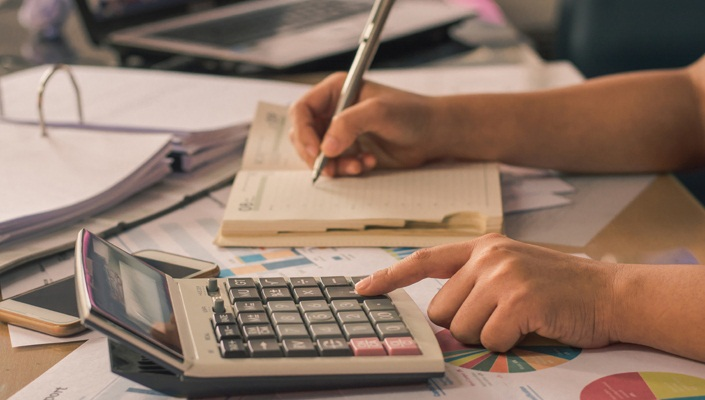You are here
Home > Education > Best Ways to Study Physics

# Best Ways to Study PhysicsFor some being good at physics comes naturally. For the rest of us, getting a good grade requires a notable amount of hard work. Luckily by mastering some foundational skills and practicing often, almost anyone can learn physics material. A better understanding of physics sheds light on some of the seemingly mysterious forces that govern the way the world works. Here, we have discussed a few ways to help you study physics in the best way possible.

### Memorize basic equation and constants

In physics, specific forces such as the acceleration due to gravity are assigned a mathematical constant. This is another way of indicating that these forces remain the same irrespective of where or how they are used. It is a smart idea to memorize some of the universal constants. Most often, in physics, the relationships between different forces are expressed in the form of an equation. Having the simplest equations memorized and knowing how to use them is critical when tackling both simple and complex problems.

### Study the basic derivation

Memorizing simple equations is one thing — understanding how or why these equations work is another thing. If you can learn how each basic physics equation is derived. This gives you a much more precise understanding of the relationship between the equations and makes you a more accomplished problems-solver. For example, rote learning the derivation of Gauss law will only give you surface knowledge. But understanding the derivation in depth will help you use the law in solving critical problems.

### Use Correct Units for every Problem

Using incorrect units will easily cost you marks. To get credited full marks, make sure to label your answers with correct units. Some of the most common units used for measurements in physics are given below:

• Energy/Work: Joules/kilojoules
• Force: Newtons
• Mass: kilograms
• Power: Watts

### Review Your Notes At Home

To polish your physics knowledge, take a few moments to review your notes as soon as you get home. The longer you take to review your notes, the more difficult it will be to remember, and they will seem more “foreign” to you, so be proactive and cement your knowledge by reviewing your notes daily at home.

These were a few pointers to help you succeed in your physics exam. Choose what suits you the best and start preparing for exams. All the best!

Meanwhile, you can subscribe to BYJU’S YouTube channel and learn concepts like the moment of inertia formula with the help of interactive videos. You can also have a glimpse of how BYJU’S is changing the way students learn.